# HBSE 10th Class Science Important Questions Chapter 5 Periodic Classification of Elements

Haryana State Board HBSE 10th Class Science Important Questions Chapter 5 Periodic Classification of Elements Important Questions and Answers.

## Haryana Board 10th Class Science Important Questions Chapter 5 Periodic Classification of Elements

Question 1.
How many elements are known to man? Why did he classify them?
1. Today, man has discovered 118 elements.
2. All the elements have different properties. These elements are either used individually or in combination with other elements to form an endless variety of substances.
3. Classifying the elements help us to understand their properties and produce various products.Question 2.
Explain Dobereiner’s classification of elements or Dobereiner’s triads.
1. In 1817, German chemist Johann Wolfgang Dobereiner started classifying elements on the basis of their chemical properties.
2. He discovered that there exists several ‘triads’ or ‘groups of three elements each’ that appeared to have similar chemical properties.
3. Law of Triads: If three elements are arranged in the increasing order of their atomic masses, the atomic mass of the intermediate (i.e. second) element would be almost equal to the average of atomic masses of first and third elements. This is known as Law of Triads. It was given by Dobereiner.

Based on this principle he identified the following three triads:

• Lithium, Sodium and Potassium,
• Chlorine, Bromine and Iodine,
• Calcium, Strontium and Barium.

Example:

• Atomic mass of Lithium (Li) is 6.9 u, Sodium (Na) is 23.0 u and that of potassium (K) is 39.0 u.
• Here, the atomic mass of sodium is the average of lithium and potassium i.e. = ((6.9 + 39)/2) = 23. Hence, these three elements form a triad.Question 3.
The elements in the Dobereiner’s table were arranged in such a manner that the atomic mass of the intermediate (i.e. second) element would be almost equal to the average of atomic masses of first and third elements. Demonstrate this with the help of Dobereiner’s triads.
1. Table given below shows Dobereiner’s triacis.
2. We can see that in all these three triads, the atomic mass of the second element is equal or nearly equal to the average of atomic mass of first and third element.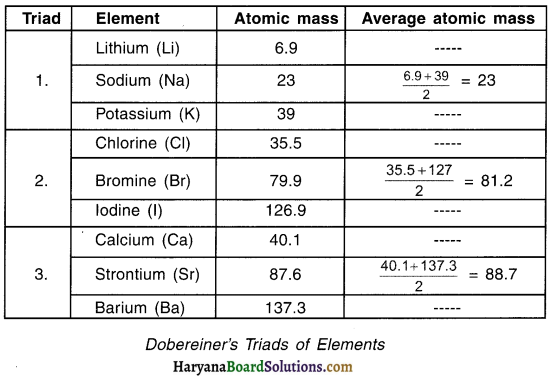Question 4.
State the limitations of Doberelner’s classification of elements.
Limitation of Dobereiner’s classification :

• Under Dobereiner’s classification, overall only a limited number of elements could be classified into triads.
• After arranging the elements in triads, it was found that there were certain other elements which could not be classified by Dobereiner’s method.

Question 5.
Explain Newlands’ method of classifying elements (or Newlands’ Law of Octaves)
Newlands’ Law of Octaves :
1. When elements are arranged in the increasing order of their atomic masses, properties of every 8th element are found to be similar to the properties of the first element.
2. In 1866, a scientist named John Newlands arranged the elements in the increasing order of their atomic masses.
3. Newlands was the first person to arrange elements in their increasing order of atomic masses.
4. During this arrangement, he found properties of every 8th element to be similar i.e. property of 1st and then 8th element would be similar. Similarly property of 2nd and 9th element would be similar and so on.
5. He called this periodicity pattern as the Law of Octaves (octaves = eight). The law can be compared with the octaves or say 8 notes found in music where in the 8th note is similar to the 1st note.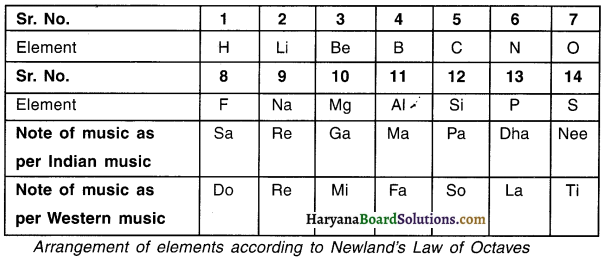Question 6.
Discuss limitations of Newlands’ Law of Octaves. OR How can you say that Newlands’ table worked well with lighter elements only?
Limitation of Newlands’ Law of Octaves:
(1) The law of octaves was applicable only upto calcium. After calcium, every 8th element did not possess properties similar to that of 1st
(2) Newlands thought that there were only 56 elements in nature. He also thought that no more elements would be discovered in the future. However, later, several new elements were discovered that could not be arranged in the table as per Newlands’ law.
(3) In order to fit elements any how into his table, Newlands adjusted two elements in the slot even if the properties of elements did not match with other elements.Question 7.
How did Mendeleev drill to arrange the elements into his Periodic Table? OR How did Mendeleev construct his Periodic Table?
When in 1869, Russian chemist Dmitri Ivanovich Mendeleev started his work of classifying elements, 63 elements were known to man.
Mendeleev started by examining the relationship of
(a) ‘Atomic mass of an element with
(b) ‘Physical property of the element’ and
(c) Chemical property of the element.

(i) Classification on the basis of chemical properties:

• For classifying the elements on the basis of chemical properties, Mendeleev started studying the compounds that a particular element formed with oxygen as well as hydrogen.
•  A compound formed when an element combines with oxygen is called oxide whereas with hydrogen is called hydride. (For example, CaO, NaH, K2H, etc.) The formulae of such oxides and hydrides were taken as ‘one of the basis of classification’.
• Mendeleev selected hydrogen and oxygen because they are very reactive and form compounds with most elements.

(ii) Classification on the basis of atomic mass:

• Mendeleev took 63 cards (1 card per element) and on each card he wrote the ‘atomic mass’ as well as ‘properties’ of a particular element.
• Mendeleev arranged the cards in the increasing order of atomic masses of elements starting from atomic number 1 to 63. Then he put the cards that showed similar properties into single group. This way he arranged elements into 8 groups. Each of these 8 groups were further divided in two sub-blocks, A and B.
• Hence, while arranging the elements in the increasing order of their atomic masses, he also arranged them in groups on the basis of their chemical properties.
• Through this classification, he concluded that “The properties of elements are periodic function (i.e. periodic in nature) of their atomic mass.” This law came to be known as Mendeleev’s Periodic Law.Mendeleev’s observation:

1. Mendeleev observed that most of the elements got a place in his periodic table.
2. The elements got arranged in the order of increasing atomic masses.
3. Elements having similar physical and chemical properties occur periodically.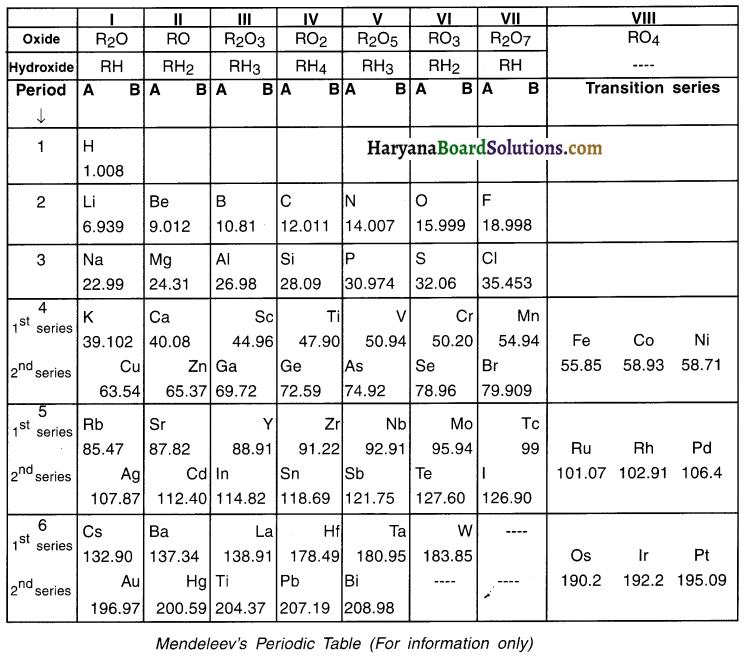Question 8.
What observations did Mendeieev make while classifying the elements into his Periodic Table?
Mendeleev’s observation:

1. Mendeleev observed that most of the elements got a place in his periodic table.
2. The elements got arranged in the order of increasing atomic masses.
3. Elements having similar physical and chemical properties occur periodically.Question 9.
Which criteria did Mendeleev use for developing his periodic table?
Criteria used by Mendeieev for developing periodic table:

• The properties of elements are the periodic function of their atomic masses. Hence, arranging elements in the increasing order of their atomic masses.
• Elements with similar properties are arranged in the same group.
• The formula of oxides and hydrides formed by an element.Question 10.
Discuss the anomalies (irregularities) of Mendeleev’s Periodic Table.
Anomalies (irregularities) of Mendeleev’s Periodic Table:
(1) Sequence of few elements was inverted:

•  In Mendeleev’s table, the elements were arranged in increasing order of their atomic masses. However, at few instances Mendeleev did not follow this arrangement.
• At few places he placed elements with slightly higher atomic mass before elements with lesser atomic mass. This means he inverted his sequence. He did this so that elements with similar properties could be grouped together.

(2) Gaps were kept at few places:

• Mendeleev had left some blank spaces in his Periodic Table. He was very sure that there exists some elements that would fit these spaces.
• Mendeleev named those blank places with a Sanskrit numeral prefix called ‘Eka

Example:
‘Eka’ means one place below something. So, Eka-aluminium means one space (left blank) below aluminium. In this manner scandium (Sc), gallium (Ga) and germanium (Ge) discovered later had properties similar to Eka-boron, Eka-aluminium and Eka-silicon respectively.

Question 11.
Discuss the achievements of Mendeleev’s Periodic Table.
Achievements of Mendeleev’s Periodic Table:
(1) The gaps that Mendeleev had left in his table got properly filled when new elements were discovered.
(2) Chemists not only accepted Mendeleev’s table but also conferred him as the ‘originator of the concept’ on which Modern Periodic Table is based.
(3) Noble gas elements such as helium (He), neon (Ne), etc. were not known during Mendeleev’s time. So, those elements were not present in his table. But, Mendeleev’s table was so precisely designed that when these gases were discovered they were easily placed in a new separate group without disturbing the existing order.Question 12.
Elements in Mendeleev’s Periodic Table were arranged in increasing order of their atomic masses.
Then why did Mendeleev place cobalt Co (atomic mass 58.93) before element nickel Ni (atomic mass 58.71) when In fact atomic mass of nickel was lesser than cobalt?
1. Although mass of cobalt (58.93) was slightly more than Nickel (58.71), even then cobalt was placed before Nickel. Mendeleev did this so that he could maintain the properties of element placed in one group.
2. The properties of Co, Rh and Ir are similar and that of Ni, Pd and Pt are similar. Hence, Mendeleev placed Co before Ni so that elements Co, Rh and Ir having similar properties could be arranged in the same group.

Question 13.
Compare the properties of Eka-aluminium and gallium to sho the brilliant prediction Mendeleev’s table had.
1. During Mendeleev’s time, gallium was not discovered. But, Mendeleev proposed properties of elements that could be filled in blank spaces that he left in his table. One such element was eka-aluminium.
2. The properties given by Mendeleev for Eka-aluminium and the actual element gallium that later replaced Eka-aluminium were quite similar. This can be seen in the table below.

Question 14.
Why did Mendeleev leave gaps in the Periodic Table?
Gaps were kept at few places:

• Mendeleev had left some blank spaces in his Periodic Table. He was very sure that there exists some elements that would fit these spaces.
• Mendeleev named those blank places with a Sanskrit numeral prefix called ‘Eka

Example:
‘Eka’ means one place below something. So, Eka-aluminium means one space (left blank) below aluminium. In this manner scandium (Sc), gallium (Ga) and germanium (Ge) discovered later had properties similar to Eka-boron, Eka-aluminium and Eka-silicon respectively.

Question 15.
Discuss limitations of Mendeleev’s classification.
Limitation of Mendeleev’s classification:
(1) Position of hydrogen:
Mendeleev had placed hydrogen (H) element in group 1 i.e. group of alkalis. He did so because like alkali metals, hydrogen combines with halogens and oxygen and sulphur to form compounds having similar formulae.

Contrary to this hydrogen also showed properties similar to elements of group 17 i.e. group of halogens. Thus, hydrogen could be placed in group 17 as well. Due to this confusion hydrogen could not be assigned a fixed place in Mendeleev’s Periodic Table.(2) Position of isotopes:

• Isotopes are atoms of the same element having similar chemical properties but different atomic masses.
• Several elements have isotopes. For example, hydrogen has three isotopes and all have separate masses. Even then all these three were placed in one single position as hydrogen element H.

(3) Wrong order of some elements:

Mendeleev had arranged the elements on the basis of increasing order of atomic masses however, he broke this rule for certain elements. For example. he placed cobalt (Co) having larger mass before nickel (Ni) having lesser mass. This made it difficult to predict how many elements can be discovered between such two elements.

Question 16.
State the three limitations of Mendeleev’s Periodic Table.
(1) The position of hydrogen could not be correctly assigned.
(2) The table could not assign proper position for the isotopes of various elements.
(3) Some elements were not arranged on the basis of their increasing atomic mass. This posed a question as to how many elements could still take the place between two elements.

Question 17.
What is a periodic table?
Periodic table:

• The Periodic Table is a chart in which all the elements known to us are arranged in a systematic manner.
• A Periodic Table is divided into rows (periods) and columns (groups).
• Elements having similar properties are placed in the same group (i.e. vertical column).

(For Information only: Why is Periodic Table called so?

• During classification of elements it was found that the elements show similar physical and chemical properties after a fix interval or say ‘period’ i.e. the elements are periodic in their nature.
• The table was then prepared based on the periodic nature or periodicity of elements and so the table is called Periodic Table.)

Question 18.
How was Periodic Law of Mendeleev Improved Into Modern Periodic Law?
1. Mendeleev had arranged the elements in his table on the basis of increasing atomic masses. In 1913, Henry Moseley showed that, rather than ‘atomic mass’, ‘atomic number’ is a ‘better fundamental property’. Henry said, atomic number and not atomic mass determine the chemical properties of elements.
2. Looking to the facts, the Periodic Law given by Mendeleev was modified into Modern Periodic Law which states “Properties of elements are a periodic function of their atomic number”.

Question 19.
State Mendeleev’s Periodic Law and Modern Periodic Law.
(a) Mendeleev’s Periodic Law:
Properties of elements are the periodic function of their atomic masses.

(b) Modern Periodic Law:
Properties of elements are a periodic function of their atomic numbers.

Question 20.
On what basis were the elements arranged in the Modern Periodic Table? Why?
1. The elements in the Modern Periodic Table were arranged on the basis of Modern Periodic Law which states that elements are a periodic function of their atomic numbers.
2. Atomic number (Z) tells us the number of protons in the nucleus of the atom of an element.
3. This number i.e. the atomic number goes on increasing as one moves from one element to the next.
4. Thus, in Modern Periodic Table the elements were arranged in the increasing order of their atomic number Le. Z.Question 21.
Discuss the arrangement of elements In groups and periods in Modern Periodic Table.
The Modern Periodic Table has 18 vertical columns known as ‘groups’ and 7 horizontal rows known as ‘periods
(a) Placement of an element in a group:
(i) Elements are placed in a particular group on the basis of the ‘valence electrons’ i.e. elements having same number of valence electrons will be placed same group.

Example:

• The electronic configuration of fluorine (f) is 2, 7 and that of chlorine (Cl) is 2, 8, 7. This means both these elements have valency = 1. Hence, both these elements are placed in same group i.e. group no. 17.
• Note that as we go down in a group, the number of shells increase. Fluorine has only 2 shells whereas chlorine has 3.

(b) Placement of an element In a period:
(i) Within a horizontal period, as one moves from left to right, the ‘elements have same number of shells’ but, ‘different valence electrons
(ii) Moreover, on moving left to right, the number of electrons of valence shell increase by 1 unit because the elements are arranged in the increasing order of their atomic number by 1 unit.
(iii) Thus, elements having same number of shells are placed in the same period. For example, Na, Mg, Al, Si, etc. have 3 shells and hence are placed In the 3rd period.

Question 22.
How are electrons arranged in various shells? How do we determine the number of elements in each period on the basis of number of elements in a shell?
Arrangement of electrons in a shell:
1. The maximum number of electrons that can be accommodated in a shell depends on the formula 2n2 (where n = the number of given shells from the nucieusý’
2. Letter ‘K’ denotes the first shell, ‘L’ second, M’ third and so on. Thus for shell ‘K, n = 1, for ‘L, n = 2 and for ‘M’, n = 3.
3. By knowing the values of ‘n’ for K, L and M we can derive the number of electrons that each of these shells can hold. It is:

• K shell = 2(n)2 = 2(1)2 = 2 electrons
• L shell = 2(n)2 = 2(2)2 2(4) = 8 electrons
• M shell = 2(n)2 = 2(3)2 = 2(9) = 18 electrons

4. Based on this calculation we say that the 1st period has 2 elements and period has 8 elements.
5. Although M shell can hold 18 electrons but third period can hold only 8 electrons. Therefore, 3 period can hold only 8 elements.Question 23.
What do you mean by periodic properties?
1. The properties which are determined by the electronic configuration of elements or which depend on the electronic configuration of elements are known as periodic properties.
2. Also, the properties which show a recurring gradation within the same group or along a period are known as periodic properties.
Example: Valency, atomic radii (atomic size), metallic property, electronegativity, etc. exhibit periodic properties.

Question 24.
Bring out the trends of change In valency, atomic size and metallic and non-metallic properties in groups and periods.

 Characteristic Groups Periods (1) Valency All the elements of a group have same valency. On moving from left to right, the valency of elements increase from 1 to 4 and then goes on decreasing to 0 (zero). (2) Atomic size (Radius of atom) As we move down in a group, the size of atoms i.e. atomic size increase On moving from left to right in a period, the size of the atoms decrease. (3) Metallic and Non-metallic properties Going down in the group, the metallic property of elements increase On moving left to right in a period, the metallic property of elements decreases while non-metallic property increases.

Question 25.
What is valency? How is it calculated?
1. Relative ability of an element to combine with other element is known as a valency. OR Valency is the combining capacity of an atom of an element to acquire noble gas configuration.
2. Valency depends on the number of valence electrons that an atom of an element has.
3. The valency of an element is

• Either equal to the number of electrons in the valence shell OR
• Equal to eight minus the number of electrons in the valence shell.

Question 26.
Explain the trend of valency within a period and a group. OR How does the valency vary in a period on going from left to right and while going down In a group?
1. As you move in the period from left to right, the valency first increases from 1 to 4. Then it decreases from 4 to 0.
2. All the elements in a given group possess equal number of electrons. Hence, valency does not change within a group.

Question 27.
Atomic size:

• The radius of the atom is called the atomic size of the atom.
• Atomic size (or radius) can be visualized as the distance between the centre of the nucleus and the outermost shell of an isolated atom.
• Atomic radius is expressed in angstrom (A°), or centimeter (cm) or picometer (pm). 1 pm = 10 12m.
• For example, the atomic radius of hydrogen atom is 37 pm.

Question 28.
Why does atomic radii increase as we go down in a group, while decrease as we move from left to right in a period? OR Explain the trends in atomic size of elements In a period and a group.
Situation in a group :
1. In a group as we move from top to down, new orbits get added in the elements.
2. For example, in the first group, Lithium (Li) has 2 orbits, Sodium (Na) has 3 orbits, Potassium (K) has 4 orbits and so on.
3. Since the orbits increase as we move down, naturally their radii also increase.

 Elements of first group No. of orbits Atomic Radius (pm) Li Na K Rb Cs 2 3 4 5 6 152 186 231 244 262

Situation in a period :

• In a period, as we move form left to right, no new orbits are added unlike in group.
• Secondly, in the period, the positive electric charge of nucleus attracts more electrons and so the radii of atoms decrease.
• Thus, as we move from left to right in a period, the atomic radii decreases.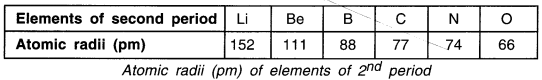Question 29.
Explain the trends of the metallic character in a period and a group.
Trend of the metallic character in a group:
On moving down in a group, the effective nuclear charge experienced by valence electrons decreases because the outermost electrons are far away from the nucleus. Therefore, tendency of the element to lose electrons increases and electrons can be lost easily. Hence, the metallic character increases on moving down in a group.

Trend of the metallic character In a period:

• On moving from left to right in a period the effective nuclear charge experienced by valence electrons increases.
• Therefore, the tendency to lose electrons will decrease.
• Thus metallic character decreases in a period on moving from left to right.

Question 30.
Explain the trends of the non-metallic character in a period and a group.
Trend of the non-metallic character in a group:
On moving down within a group, the non-metallic character decreases.

Trend of the non-metallic character in a period:

• On moving from left to right in a period, the nuclear charge experienced by valence electrons increases. So, the tendency to attract electrons increase.
• As a result, the non-metallic character increases in a period on moving from left to right.

Question 31.
What are metalloids or semi-metallic elements? Give example.
1. Elements which possess properties of both metals and non-metals are known as metalloids or semi-metallic elements.
2. In the Modern Periodic Table, a zig-zag line separates metals and non-metals. The border line elements such as boron (B), silicon (Si), germanium (Ge), arsenic (As), antimony (Sb), tellurium (Te) and polonium (Po) on this zig-zag line are known as metalloids or semi-metals.

Question 32.
Give difference between ‘Elements of a group’ and ‘Elements of a period’

 Elements of a group Elements of a period 1. The atomic number of elements increases as we move down within a group. 1. The atomic number of elements increases on moving from left to right within a period. 2. All the elements in a group have same number of valence electrons. 2. The number of valence electrons of the elements in a group increases. 3. Elements within a group possess similar chemical reactivity. 3. Elements within a group do not possess similar chemical reactivity. 4. The atomic radius and metallic character increase on moving down the group. 4. The atoms radius and metallic character decrease on moving from left to right within a period.

Question 33.
1. The method of classifying elements on the basis of triads was given by Dobereiner.
2. Under this method, Dobereiner stated that if three elements having similar chemical properties are arranged in their increasing order of atomic masses, the atomic mass of the intermediate (second) element would be similar to the average of atomic masses of first and third element.
3. However, under this classification, overall only a limited number of elements could be classified and so this method failed later on.
4. It was also discovered that more elements could be added to these triads.
5. For example, fluorine could be added to the triad of chlorine, bromine and iodine. Similarly magnesium could be added to the triad of calcium, strontium and barium.
6. Hence, scientists thought that rather than classifying elements only in triads, perhaps they could be arranged in larger groups.

Question 34.
Newlands’ law of arranging elements came to be known as ‘Law of Octaves’. Give reason.
Newlands’ Law of Octaves :
1. When elements are arranged in the increasing order of their atomic masses, properties of every 8th element are found to be similar to the properties of the first element.
2. In 1866, a scientist named John Newlands arranged the elements in the increasing order of their atomic masses.
3. Newlands was the first person to arrange elements in their increasing order of atomic masses.
4. During this arrangement, he found properties of every 8th element to be similar i.e. property of 1st and then 8th element would be similar. Similarly property of 2nd and 9th element would be similar and so on.
5. He called this periodicity pattern as the Law of Octaves (octaves = eight). The law can be compared with the octaves or say 8 notes found in music where in the 8th note is similar to the l note.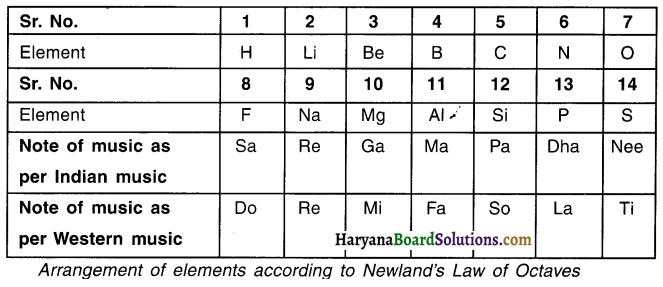Question 35.
Differentiate between groups and periods of Modern Periodic Table.

 Groups Periods 1. The vertical columns in the Periodic Table are called groups. The horizontal rows In the Periodic Table are called periods. 2. There are 18 groups. There are 7 periods. 3. Elements of a given group have similar chemical properties. With changing period, the chemical properties of elements in the period also change. 4. Through group number we can find out the number of electrons in the outermost orbit of an atom of an element. Through period number we can find out the number of orbits of atoms.

Question 36.
Two elements X and Y belong to groups 1 and 3 respectively In the same period. Compare these elements with respect to their —
(a) Metallic character (b) Size of atoms (c) Formulae of their oxides and chlorides
(a) As one moves in a period from left to right, the metallic character decreases. Hence, the metallic character decreases while moving from X to Y.
(b) As one moves in a period from left to right, the atomic radius decreases. Hence, atoms of element Y are smaller than that of element X.
(c) Oxides of element X and Y: X2O, X2O3, Chlorides of element X and Y: XCl, YCl3

Question 37.
Atomic number of magnesium is 12. What information can you get from this? (Hint: Keep In mind the Modern Periodic Table.)
1. The atomic number of magnesium is 12. So, its electronic configuration is (2, 8, 2).
2. From this, we can say that it is positioned in the third period arid second group in the Periodic Table.
3. There are 3 orbits (2, 8, 2) and there are 2 electrons in its outermost orbit.
4. It has 2 electrons in the last orbit and so it will lose these 2 electrons and hence become mg2+ ion. Thus, its vaiency is +2.

Question 38.
Elements of which groups of the Periodic Table can easily lose electrons and elements of which groups of the Periodic Table can easily gain electrons?
1. Elements of group IA, IIA, IIIA and that of group IB. IIB and IIIB will easily lose electrons.
2. Elements of group IVA, IVA, VIIIA and VIIIB will neither lose nor gain electrons.
3. Elements of group VA, VIA, VIIA and that of group VB, VIB and VIIB will easily gain electrons.

Question 39.
The position of three elements A, B and C in the Modern Periodic Table is shown below: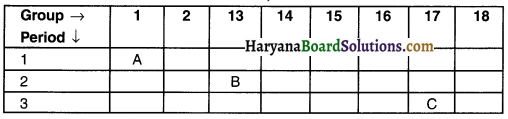Giving reason for the following statements in one or two sentences.
(a) Element A is a metal.
(b) Atom of element C has a larger size than atom of element B.
(c) Element B has a valency of 3.
(a) Element A has 1 valence electron. So it can lose this electron and become electropositive. Hence, we can consider element A as metal.
(b) Element B belongs to 2nd period and so has 2 shells whereas element C belongs to 3rd period and so it has 3 shells. As a result, atoms of element C are bigger than atoms of element B.
(c) Element B belongs to group 13 and so its valency is 3.Question 40.
The atomic number of elements A,B, C, D and E are given below: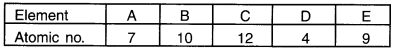From the above table, answer the following questions:
(a) Which two elements are chemically similar? (b) Which is an inert gas? (c) Which elements belong to 31 d period of Periodic Table? (d) Which element among these is a non-metal?
(a) Element C and D have similar electronic configuration, i.e. 2, 8, 2 and 2, 2. Hence, these two elements are similar.
(b) Electronic configuration of element B is 2, 8. Hence, it has 8 electrons in its outermost shell. So, element B is an inert gas.
(c) Atomic number of C is 12 and so its electronic configuration is (2, 8, 2) i.e. it has 3 shells. Hence, element C belongs to 3rd period of the Modern Periodic Table.
(d) Element A (2, 5) is non-metal.

Question 41.
What is meant by periodicity? Why are the properties of elements in the same group similar?
1. The properties of the elements n the Modern Periodic Table depend on regular changes that are seen in the electronic configuration of elements arranged in groups and periods. This law is known as periodicity.
2. Valency decides the properties that an element possesses.
3. The electronic configuration of the valence orbit of all the elements in a group is same.
4. Thus, in the same group, properties of an element remains same.

Question 42.
The electronic configuration of an element ‘X’ is 2, 8, 8, 2. To which (a) period and (b) group of the Modern Periodic Table does ‘X’ belong? State its valency. Justify your answer in each case.
(a) The electronic configuration of element X states that the element has four shells namely K, L, M and N. Hence, element X belongs to 4th period.
2. There are 2 electrons in the outermost i.e. N shell and so element X belongs to group 2.

Question 43.
Atomic number is considered to be a more appropriate parameter than atomic mass for classification of elements in a periodic table. Why?
1. Atomic mass does not properly tell us the properties of elements. Moreover, the elements cannot be arranged in the increasing order of atomic mass because of certain anomalies.
2. The properties of elements depend upon the number of electrons present in the valence shell. The number of electrons can be known by its atomic number. In this regard, an element can be classified in a better way through atomic number.
3. Hence, atomic number is considered to be a more appropriate parameter than atomic mass for classification of elements in the Periodic Table.Question 44.
From the part of a Periodic Table, answer the following questions: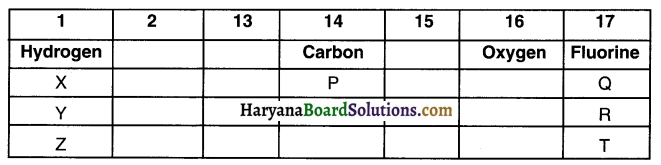(a) Atomic number of oxygen is 8. What would be the atomic number of Fluorine?
(b) Out of ‘X’ and ‘Q’ which element has larger atomic size. Give reason for your answer.
(c) Out of ‘Y’ and ‘Z’ which element has smaller atomic size. Give reason for your answer.
(a) In the Modern Periodic Table, elements are arranged on the basis of increasing order of their atomic numbers. Fluorine comes after oxygen and so atomic number of fluorine is 9.
(b) As one moves from, left to right in a period, the atomic size of the element goes on decreasing. Hence, atomic size of element Q is smaller than that of element X.
(c) As one moves down from one period to another, the numbers of shells increase. Element Y lies above element Z. So, element Y will have lesser shells than Z and hence Y’s atomic size will be smaller.

Question 45.
The atomic number of an element ‘X’ is 20. Write —
(a) Its valency
(b) Whether it Is a metal or non-metal
(c) The formula & compound formed when the element ‘W reacts with an element ‘Y’ of atomic number 8.
(a) Since the atomic number of X is 20, its electronic configuration will be 2, 8, 8, 2. Hence, its valency is 2.
(b) Its valency is 2, so it belongs to group 2 i.e. group of metals. Moreover, since its valency is 2. it will lose 2 electrons which means it shows the property of metal.
(c) Atomic number of Y is 8. So its electronic configuration is (2, 6). In other words, valency of Y is 2. Moreover valency of X is also 2. So, the compound formed by sharing 2 electrons will be compound ‘XV’.

Question 46.
An element X belongs to 13th group of periodic table. State its valency. What will be the formula of its sulphate?
Element X belonging to group 13th has 3 valence electrons and so its valency is 3. Sulphate of X = X2(SO4)3.

Question 47.
The elements Li, Na and K, each having one valence electron, are in period 2,3 and 4 respectively of Modern Periodic Table.
(a) In which group of the periodic table should they be?
(b) Which one of them is least reactive?
(c) Which one of them has the largest atomic radius? Give reason to justify your answer in each case.
(a) All these elements have 1 valence electron and so they belong to Group 1.
(b) Li is the least reactive. Since Li is 2nd period it has only 2 shells. So, its outermost orbit is very near the nucleus. As a result, is difficult to remove the electron. This makes it least reactive of the three elements.
(c) Element K lies in 4th perioded has 4 shells. Hence, it has largest atomic radius of the three.

Question 48.
Sudha madam asked students to place three elements namely lithium, sodium and potassium in one group or say triad. Now, answer the following questions.
(a) How could she place all the three elements in one group?
(b) What is the name of this group? State the properties of the elements of this group.
(a) Sudha madam placed the three elements in the same group because all three elements have similar properties.
(b) The name of this group is ‘alkali metal group’. Properties of the elements of this group are-

• All these elements are metals.
• Valency of each element is 1.
• These metals readily react with water and form alkalis and hydrogen gas.Question 49.
In the Science class this morning, Prakash sir taught students how Newlands classified the elements known to him. He then gave the students an assignment to role-play Newland. Prakash sir gave them some elements along with their atomic weights which are as follows.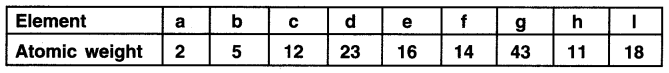(a) Which two elements will have similar properties on the basis of Newland’s law of octaves? Justify the reason for your selection.
Newlands had arranged the elements in the increasing order of their atomic weights. So, first we arrange the elements.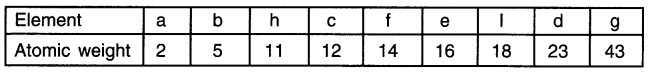As per Newland’s law of Octaves, if elements are arranged in the increasing order of the atomic weights then every 8th element will have properties similar to the just one. Hence, element ‘a’ having atomic mass 2 will have properties similar to element ‘d’ having atomic mass 23. Similarly, element ‘b’ and ‘g’ will can be grouped together.

Question 50.
Introduction to the groups of elements of the Modern Periodic Table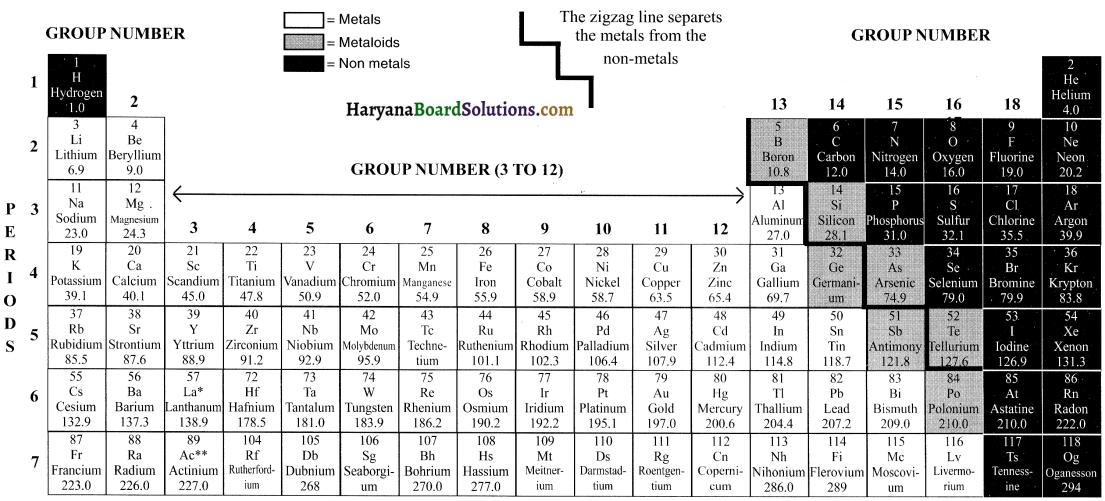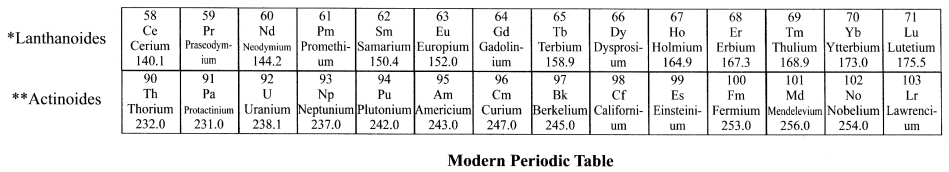Introduction:

118 elements are known to us. Majority of these elements are metals. These metals are not very reactive. They are malleable and ductile and good conductors of heat and electricity. The remaining elements are non-metals and semi-metals (metalloids).

Brief explanation of each group of the Modern Periodic Table is as follows:

(1) Group 1 elements (Alkali metals): Elements of this group are known as ‘Alkali metals’. There are in total 6 alkali metals from Lithium to Francium. Alkali metals are very soft and highly reactive metals. They are so reactive that they have to be stored in substances like oil because if kept open they will start reacting with atmospheric gases.

If they are not found freely then how do we obtain them? Well, chemists extract them from their compounds. Can explode when exposed to air The metals are malleable, ductile and good conductors. They have only 1 valence electron. They are always eager to lose this 1 electron and join with other electron to form a positive ion or say cation.

(2) Group 2 elements (Alkaline earth metals): Elements of this group are known as ‘alkaline earth metals’. There are in total 6 elements from Beryllium to Radium. Alkaline metals are also reactive metals but not as reactive as alkali metals. They have 2 electrons in their outer shell. So, they tend to lose 2 electrons to form positive ions.

(3) Group 3 to Group 12 (Transition metals (elements)): Elements of this group are known as ‘Transition metals’. They are moderately reactive. They are malleable and ductile. They have high melting and boiling points

(4) Group 13 to 16: Group 13 to 16 contains a mix of metals, metalloids and non-metals.

Metalloids: Metalloids are elements that have few properties of metals and few of non-metals.
Metalloids are the smallest class of elements (the other two classes of elements are metals and nonmetals). There are just seven rnetalloids namely boron (B), silicon (Si), germanium (Ge), arsenic (As), antimony (Sb). tellurium (Te) and polonium (PO).

(5) Group 17 (Halogens): Halogens are non-metals. Examples of halogens are Fluorine (F), chlorine (Cl), Bromine (Br), Iodine (I), etc. The term “halogen” means salt-former” and compounds containing halogens are called “salts”.All halogens have 7 electrons in their last shell. So, they gain 1 electron They are highly reactive with alkali metals (group 1) arid alkaline metals (group 2).(6) Group 18 (Noble gases): The 18th group (extreme right group) consists of non-metal elements known as noble gases or inert gases. The valence (outermost) shells of all noble gases are completely filled with electrons (2 for Helium,8 for all others). Hence, the noble gases are very stable and extremely less reactive.There are 6 noble gases that occur naturally. They are helium (He), neon (Ne), argon (Ar), krypton (Kr), xenon (Xe), and the radioactive radon (Rn).

(7) Inner-transItion metals: Inner-transition metals are the elements listed below the Modern Periodic Table. These are 30 rare earth metals.

Why are they placed below the table and not In the table?
If you look at the Modern Periodic Table, you will notice that after the element Lanthanum (atomic number 57), the next element that appears is Hafnium (atomic number 72). This means that the elements with atomic number 58 to 71 are missing. Similarly, after actinium (atomic number 89) the next element that occurs is Rutherfordium (atomic number 104).

The missing elements having atomic number 58 to 71 are placed below the table and are called lanthanides because they exhibit properties similar to element Lanthanum (atomic number 57). Similarly, the missing elements having atomic number 90 to 103 are placed below the table and are called actinides because they exhibit properties similar to element actinium (atomic number 89)

The inner-transition metals were discovered quite recently. Scientists and chemists are yet to determine their exact characteristics and properties. Hence, it was decided to keep these elements separate below the Modern Periodic Table.

(8) The case of hydrogen — an important point: Hydrogen is placed in group 1. However, its position is a matter of discussion. The reason for it follows.
Hydrogen shows similarity to group 1 elements i.e. alkalis as well as to group 17 elements i.e. halogens.

(a) Hydrogen’s similarity with alkalis: Hydrogen has only 1 electron in its valence shell. All the electrons that have 1 electron in their outer shells are placed in group 1 For example, lithium (2, 1), sodium (2, 8, 1) and so on. This means, hydrogen’s electronic configuration resembles that of alkali metals and hence it is placed in group 1. Hence, both alkalis and hydrogen have the tendency to lose 1 electron and become positive ion. The way alkali metals react with halogens (i.e. elements of group 17), oxygen and sulphur, hydrogen also reacts in a similar way. Hence, hydrogen is placed in the grotip of alkalies.

(b) Hydrogen’s simIlarity with halogens: The hydrogen also shows properties to halogens i.e. elements of group 17. All the elements of halogen group are 1 electron short of attaining their octet configuration. For example, Fluorine (2, 7),chlorine (2, 8, 7), etc. Hydrogen with electronic configuration of (1) is also 1 short of attaining octet configuration. So, the way the halogens require 1 electron to complete their configuration and become stable, hydrogen also require 1 electron to complete its configuration.

Hence, both halogens and hydrogen have the tendency to accept 1 electron and become negative ion. Halogens exist as diatornic molecules i.e. the molecule of halogens is made up of 2 atoms. For example, fluorine exists as, F2, chlorine as C12 and so on. Similarly, hydrogen also exists as a diatomic molecule i.e. as H2. Just like halogens, hydrogen also combines with metals and non-metals to form covalent compounds. Considering the above points we conclude that hydrogen also resembles halogens.

(c) Conclusion: Since hydrogen shows properties similar to alkanes (group 1 elements) and halogens (group 2 elements). its position in the periodic table is till date contradictory and hence not fixed. However, we place hydrogen in group I simply for the purpose of convenience (technically, it can be placed in halogen group as well.

Very Short Answer Type Question :

Question 1.
What is the need to classify elements?
We can learn a lot about the properties and characteristics of elements when they are systematically classified. Hence, there arose a need to classify them.

Question 2.
What were elements first classified into?
When elements were classified for the first time they were classified as metals and non-metals.

Question 3.
Who was Dobereiner?
John Wolfgang Dobereiner was a German chemist who in 1817 tried to arrange the elements with similar properties into groups.

Question 4.
Dobereiner identified some elements that could be grouped into groups of three on the basis of the atomic mass of elements. Such groups were called Dobereiner’s Triads.Question 5.
State the principle of Dobereiner’s Triads.
When three elements are arranged in the order of increasing atomic masses, the atomic mass of middle element is roughly the average of the atomic masses of other two elements.

Question 6.
The three elements A, B and C with similar properties have atomic masses X, Y and Z respectively. The mass of Y is approximately equal to the average mass of X and Z. What is such an arrangement of elements called as? Give one example of such a set of elements.
Such an arrangement of elements is called Dobereiner’s triads.

Example:
Lithium (ll): atomic mass = 6.9, Sodium (Na): atomic mass = 23, Potassium (K): atomic mass = 39
Atomic mass of Na =$$\frac{39+6.9}{2}$$= 23

Question 7.
Can Na, Si, Cl form Dobereiner’s triad?
Although the atomic mass of Si Is approximately the average of atomic masses of Na and Cl. still these three elements cannot form Dobereiner’s triads because the properties of these elements are different.

Question 8.
What can be considered as Newland’s biggest discovery?
Newland’s thought that on arranging elements on the basis of increasing atomic masses, every 8th element has properties similar to the 1st can be considered his biggest discovery.

Question 9.
Fill the table given below with first seven elements as arranged by Newland’s.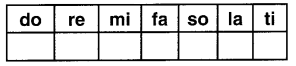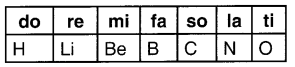Question 10.
State Newland’s Law of Octaves.
When elements are arranged in the order of increasing atomic masses, the properties of the eight elements (starting from a given element) are repetitions of the properties of the first element.Question 11.
As per Patel Sir, Newland Law of Octaves was applicable only to tighter elements. Did he pass a correct statement? Why?
Yes, Reason: Newland’s Law of Octaves is applicable only to lighter elements having mass upto element calcium. After calcium, the 1st and 8th element do not have similar properties.

Question 12.
Define Mendeleev’s Periodic Law.
The properties of elements are a periodic function of their atomic masses,

Question 13.
What was the base of classification adopted by Mendeleev?
(a) Arranging elements on the basis of increasing atomic masses.
(b) Grouping elements that have similar properties.

Question 14.
Why did Mendeleev leave some gaps in his periodic table?
Mendeleev predicted that the gaps will be occupied by elements that would be discovered in some time future.

Question 15.
Magnesium and calcium are metals. Is this enough to classity both these elements into same group? Why?
No, Reason: Elements should be put into same group on the basis of some fundamental properties that are common to such elements.Question 16.
63

Question 17.
What did Mendeleev focus on while considering chemical properties as one of the bases of classification?
While considering chemical properties as one of the bases of classification, Mendeleev concentrated on compounds that the elements formed by combining with oxygen and hydrogen.

Question 18.
Why did Mendeleev consider compounds that elements formed with hydrogen and oxygen as a base of classification?
Hydrogen and oxygen are very reactive and they form compounds with most elements. This provides a great help in classifying the elements. Hence,……….

Question 19.
Why were noble gases discovered very late?
Elements are discovered on the basis of their reactivity with oxygen. Noble gases are inert i.e, not reactive. Hence, it was quite difficult to imagine existence of such elements.

Question 20.
Why hydrogen dId not find a suitable position in Mendeleev’s Periodic Table?
Hydrogen is an element which shows properties similar to the elements of group 1 as well as to the elements of group 17 ie. halogens. Hence, till date where should hydrogen be placed is not correctly determined even in the Modern Periodic Table.

Question 21.
What are isotopes?
Atoms of an element having same atomic number but different atomic masses are called isotopes.

Question 22.
Define valency.
The combining capacity of an atom of an element to acquire noble gas configuration is called valency.

Question 23.
State Modern Periodic Law.
Properties of elements are a periodic function of their atomic number.Question 24.
What are groups in a Modern Periodic Table?
The 18 vertical columns of the Modern Periodic Table are called groups.

Question 25.
What are periods?
The 7 horizontal rows of the Modern Periodic Table are called periods.

Question 26.
Look at the table given below.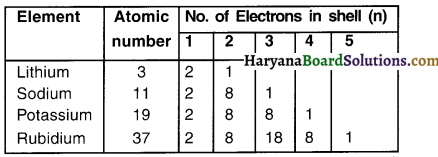To which group do these elements belong? Wily?
All these elements belong to Group 1 because every element has one valence electron in its outermost shell.

Question 27.
Loot at the table below.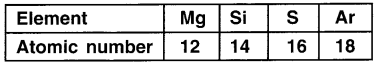State the electronic configuration of each element. To which period will these elements belong? Why?
1. Mg – 2, 8, 2, Si – 2, 8, 4, S – 2, 8, 6 Ar – 2, 8, 8
2. Electrons of all these elements are arranged in 3 shells and so all lie in 3rd period.Question 28.
An element Q lies in group 2 whereas element R lies in group 15 of the periodic table. Now fill the table.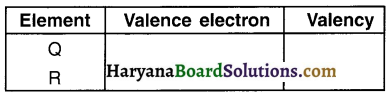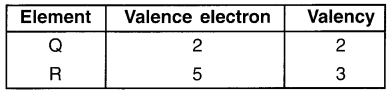Question 29.
Why all the elements in spite of belonging to one same period have different properties?
Within a period the number of valence electrons is different for each element. So, the tendency to lose or gain electrons for each element varies. Hence …………

Question 30.
The distance between the centre of the nucleus and the outermost shell of an isolated atom is called atomic radius or atomic size.

Question 31.
What are metalloids?
Elements that have some properties of metals and some of non-metals are known as metal bids.

Question 32.
State the number of valence electrons and valency of most of the elements of group 18.
Generally, the elements of group 18 have 8 valence electrons and their valency is O (zero).

Question 33.
An element Q lies in group 2 of the periodic table. State the formula of its chloride and oxide.
Chloride — XCl2; Oxide XC.

Question 34.
State the formula of the compound formed when an element X of group 14 combines with element Y of group 16.
Electronic configuration of X : 2, 8, 4
Electronic configuration of Y : 2, 8, 6
∴ X will combine with 2 atoms of Y.
∴ The formula of compound formed will be XY2.Question 35.
Write the positions of metallic, non-metallic and metalloid elements in the Modern Periodic
Table.
In the Modern Periodic Table, metallic elements are on the left side while non-metallic elements are on the right side. Metalloids or semi-elements are in the middle of the Periodic Table.

Question 36.
The construction of Modern Periodic Table owes to research of which scientist?
(A) Sir Ramsay
(B) Moseley
(C) Lord Rayleigh
(D) Mendeleev

True or False

1. ………… elements are known to us and out of them are available naturally.
118; 98

2. Elements with similar properties were arranged in groups for the first time in the year …………
1817

3. It was ………… who found that properties of elements are a periodic function of their atomic masses.
Newlands

4. As per the ‘Law of Octaves’, lithium shows properties similar to …………
Sodium

5. Newland’s Periodic Table ended at element number named ………… named …………
56; Thorium6. The main credit for classifying elements goes to …………  (write full name).
Dmitri Ivanovich Mendeleev

7. While considering chemical properties as one of the parameter for classifying the elements, Mendeleev focused on compounds that the elements formed with ……………
Hydrogen and oxygen

8. Element X has atomic number 20. It should find its place in ………… period.
Fourth

9. Element that took place of Eka-silicon was …………
Germanium

10. Atomic mass of Eka-aluminium: 68; Atomic mass of Eka-gallium……………
69.7

11. Hydrogen’s properties resemble ………… to ……….. and (Name of groups).
Alkalis; halogens

12. In 1913 …………….. showed that the atomic number of an element is a more fundamental property than its atomic mass.
Henry Moseley13. Atomic number is denoted as ……………….
‘Z’

14. The Modern Periodic Table takes care of limitations of Mendeleev’s Periodic Table. (state the number)
Three

15. With respect to group number, hydrogen can be placed in
Group number 1 and also 7.

16. The number of shells that can be accommodated in a shell can be calculated using the formula ………..
2n2

14. Atomic radius is measured in ………….
Pico meter (pm)

18. 1 pm = ………………………
10-12m

19. Noble gases are also known as ……………..
Inert gases

20. Metallic elements have …………….. electrons n their outermost orbit.
1 to 3

21. If you move from right to left in a period, the atomic radius will ……………..
Increase

22. Boron, silicon and germanium are examples of
Metalloids (or semi-metals)

23. Oxides of elements tend to be basic.
Metallic24. Non-metals are electro-
Negative

True or False

1. Triads are written in the increasing order of their atomic number. — False
2. Look at the table below.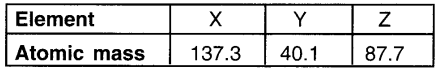Do these elements satisfy even to be a triad? (Yes = True, No = False) — True (Yes)
3. Dobereiner could identify only three triads and so his system of classification was not useful. — True
4. Newlands’ law failed after calcium — True
5. Newlands thought that after 63rd element no more elements will be discovered — False
6. Newlands put nickel and carbon in same slot — False
7. Newlands’ law worked well for heavier elements — False
8. Mendeleev knew about 7 more elements as compared to Newlands. — True
9. Nickel (atomic mass 58.93) appeared before cobalt (atomic mass 58.71) in Mendeleev’s periodic table. So as to group them together on the basis of similar properties. — False
10. Mendeleev’s Table did not consist of noble gases. — True
11. Halogens have valency of 7. — True
12. The position of hydrogen in the Modern Periodic Table is till date a matter of argument. — True
13. An element having atomic number 25.5 can be easily placed between Mn and Fe. — False
14. Each period signifies a new electronic shell getting filled. — True
15. As we go down in a group, the distance between the outermost electron and the nucleus decreases. — False
16. A zigzag line separates metals from non-metals within the Modern Periodic Table. — True
17. As the effective nucleus charge acting on the valence shell electrons increases across a period, the tendency to lose electrons decrease. — True
18. Metals are electro-positive. — TrueMatch the Following:

Question 1.

 I II 1. Dobereiner 2. Moseley a. Law of Octaves b. Triads c. Modern Periodic Table Law d. Periodic